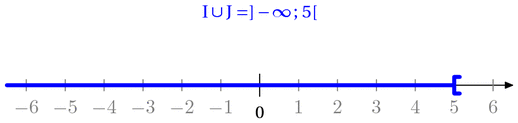Fichier f007.mp (figure 15) — Modifié le 12 Avril 2008 à 11 h 05Source

input newcourbes;
input couleur;
input geometriesyr16;
input TEX;

verbatimtex
%&latex
\documentclass{article}
\usepackage[upright]{fourier}
\usepackage{color}
\def\E{\mathrm{e}}
\let\ve\vec
\def\DR{\mathcal{D}}
\def\CR{\mathcal{C}}
\def\HR{\mathcal{H}}
\newcommand{\Mathbold}{\mbox{\boldmath$#1$\unboldmath}}
\newcommand{\pv}{\ensuremath{\: ; \,}}
\begin{document}
etex

%%%%%%%%%%%%%%%%%%%%%%%%%%%%%%%%%%%%%%%%%%%%%%%%%%%%%%%%%%%
%%%
%%                  I N T E R V A L L E S                 %
%%
%%%%%%

repere(0,0,-5,7,-1,1,.7cm,1cm);

pair A,B,C,D;
A:=r_p(-6.5,0); B:=r_p(2,0);  C:=r_p(-1,0); D:=r_p(5,0);

path d,g;

d:=(1mm,1.5mm)--(0,1.5mm)--(0,-1.5mm)--(1mm,-1.5mm);
g:=(-1mm,1.5mm)--(0,1.5mm)--(0,-1.5mm)--(-1mm,-1.5mm);

beginfig(10);
%r_axes;

drawarrow r_p(-6.5,0)..r_p(6.5,0) ;
label.bot(btex 0 etex,r_p(0,-.2));
draw r_p(0,0.2)..r_p(0,-0.2);

pickup pencircle scaled 2bp;

label.top(btex $I=]-\infty\pv 2]$ etex, r_p(-3,1) ) withcolor white;

endfig;

beginfig(11)

path d,g;

d:=(1mm,1.5mm)--(0,1.5mm)--(0,-1.5mm)--(1mm,-1.5mm);
g:=(-1mm,1.5mm)--(0,1.5mm)--(0,-1.5mm)--(-1mm,-1.5mm);

%r_axes;

drawarrow r_p(-6.5,0)..r_p(6.5,0) ;
label.bot(btex 0 etex,r_p(0,-.2));
draw r_p(0,0.2)..r_p(0,-0.2);

pickup pencircle scaled 2bp;

draw  A--B withcolor red; draw g shifted B withcolor red;  label.top(btex $I=]-\infty\pv 2]$ etex, r_p(-3,1) ) withcolor red;

%draw C--D withcolor blue; draw g shifted C withcolor blue; draw d shifted D withcolor blue;label.top(btex $J=]-1\pv 5[$ etex, %r_p(3,1) ) withcolor blue;

%draw C--B withcolor green; draw g shifted C withcolor green; draw g shifted B withcolor green;label.top(btex $I\cap J=]-1\pv %2]$ etex, r_p(1,1) ) withcolor green;

%draw A--D withcolor bleu; draw d shifted D withcolor bleu; label.top(btex $I\cup J=]-\infty\pv 5[$ etex, r_p(0,1) ) withcolor %bleu;

%r_fin;

endfig;

beginfig(12)

path d,g;

d:=(1mm,1.5mm)--(0,1.5mm)--(0,-1.5mm)--(1mm,-1.5mm);
g:=(-1mm,1.5mm)--(0,1.5mm)--(0,-1.5mm)--(-1mm,-1.5mm);

%r_axes;

drawarrow r_p(-6.5,0)..r_p(6.5,0) ;
label.bot(btex 0 etex,r_p(0,-.2));
draw r_p(0,0.2)..r_p(0,-0.2);

%pickup pencircle scaled 2bp;

draw  A--B withcolor red withpen pencircle scaled 2bp ; draw g shifted B withcolor red  withpen pencircle scaled 2bp ;  label.top(btex $I=]-\infty\pv 2]$ etex, r_p(-3,1) ) withcolor red withpen pencircle scaled 2bp;

draw C--B withcolor blue;
pickup pencircle scaled 2bp;

draw B--D withcolor blue; draw g shifted C withcolor blue; draw d shifted D withcolor blue;label.top(btex $J=]-1\pv 5[$ etex, r_p(3,1) ) withcolor blue;

%draw C--B withcolor green; draw g shifted C withcolor green; draw g shifted B withcolor green;label.top(btex $I\cap J=]-1\pv %2]$ etex, r_p(1,1) ) withcolor green;

%draw A--D withcolor bleu; draw d shifted D withcolor bleu; label.top(btex $I\cup J=]-\infty\pv 5[$ etex, r_p(0,1) ) withcolor %bleu;

%r_fin;

endfig;

beginfig(13)

path d,g;

d:=(1mm,1.5mm)--(0,1.5mm)--(0,-1.5mm)--(1mm,-1.5mm);
g:=(-1mm,1.5mm)--(0,1.5mm)--(0,-1.5mm)--(-1mm,-1.5mm);

%r_axes;

drawarrow r_p(-6.5,0)..r_p(6.5,0) ;
label.bot(btex 0 etex,r_p(0,-.2));
draw r_p(0,0.2)..r_p(0,-0.2);

pickup pencircle scaled 2bp;

%draw  A--B withcolor red; draw g shifted B withcolor red;
%label.top(btex $I=]-\infty\pv 2]$ etex, r_p(-3,1) ) withcolor red;

%draw C--D withcolor blue; draw g shifted C withcolor blue; draw d shifted D withcolor blue;
%label.top(btex $J=]-1\pv 5[$ etex, r_p(3,1) ) withcolor blue;

draw C--B withcolor green; draw g shifted C withcolor green; draw g shifted B withcolor green;label.top(btex $I\cap J=]-1\pv 2]$ etex, r_p(1,1) ) withcolor green;

%draw A--D withcolor bleu; draw d shifted D withcolor bleu; label.top(btex $I\cup J=]-\infty\pv 5[$ etex, r_p(0,1) ) withcolor %bleu;

%r_fin;

endfig;

beginfig(14)

path d,g;

d:=(1mm,1.5mm)--(0,1.5mm)--(0,-1.5mm)--(1mm,-1.5mm);
g:=(-1mm,1.5mm)--(0,1.5mm)--(0,-1.5mm)--(-1mm,-1.5mm);

%r_axes;

drawarrow r_p(-6.5,0)..r_p(6.5,0) ;
label.bot(btex 0 etex,r_p(0,-.2));
draw r_p(0,0.2)..r_p(0,-0.2);

%pickup pencircle scaled 2bp;

draw  A--B withcolor red withpen pencircle scaled 2bp; draw g shifted B withcolor red withpen pencircle scaled 2bp;  label.top(btex $I=]-\infty\pv 2]$ etex, r_p(-3,1) ) withcolor red withpen pencircle scaled 2bp;

draw C--B withcolor blue;
pickup pencircle scaled 2bp;

draw B--D withcolor blue; draw g shifted C withcolor blue; draw d shifted D withcolor blue;label.top(btex $J=]-1\pv 5[$ etex, r_p(3,1) ) withcolor blue;

%draw C--B withcolor green; draw g shifted C withcolor green; draw g shifted B withcolor green;label.top(btex $I\cap J=]-1\pv %2]$ etex, r_p(1,1) ) withcolor green;

%draw A--D withcolor bleu; draw d shifted D withcolor bleu; label.top(btex $I\cup J=]-\infty\pv 5[$ etex, r_p(0,1) ) withcolor %bleu;

%r_fin;

endfig;

beginfig(15)

path d,g;

d:=(1mm,1.5mm)--(0,1.5mm)--(0,-1.5mm)--(1mm,-1.5mm);
g:=(-1mm,1.5mm)--(0,1.5mm)--(0,-1.5mm)--(-1mm,-1.5mm);

%r_axes;

drawarrow r_p(-6.5,0)..r_p(6.5,0) ;
label.bot(btex 0 etex,r_p(0,-.2));
draw r_p(0,0.2)..r_p(0,-0.2);

pickup pencircle scaled 2bp;

%draw  A--B withcolor red; draw g shifted B withcolor red;
%label.top(btex $I=]-\infty\pv 2]$ etex, r_p(-3,1) ) withcolor red;

%draw C--D withcolor blue; draw g shifted C withcolor blue; draw d shifted D withcolor blue;
%label.top(btex $J=]-1\pv 5[$ etex, r_p(3,1) ) withcolor blue;

%draw C--B withcolor green; draw g shifted C withcolor green; draw g shifted B withcolor green;label.top(btex $I\cap J=]-1\pv %2]$ etex, r_p(1,1) ) withcolor green;

draw A--D withcolor bleu; draw d shifted D withcolor bleu; label.top(btex $I\cup J=]-\infty\pv 5[$ etex, r_p(0,1) ) withcolor bleu;

%r_fin;

endfig;

beginfig(16)

pair A,B,C,D;
A:=r_p(-3,0); B:=r_p(3,0);  C:=r_p(-6.5,0); D:=r_p(1,0);

path d,g;

d:=(1mm,1.5mm)--(0,1.5mm)--(0,-1.5mm)--(1mm,-1.5mm);
g:=(-1mm,1.5mm)--(0,1.5mm)--(0,-1.5mm)--(-1mm,-1.5mm);

%r_axes;

drawarrow r_p(-6.5,0)..r_p(6.5,0) ;
label.bot(btex 0 etex,r_p(0,-.2));
draw r_p(0,0.2)..r_p(0,-0.2);

pickup pencircle scaled 2bp;

draw  A--B withcolor vert_e; draw g shifted B withcolor vert_e; draw g shifted A withcolor vert_e;
label.top(btex $I=]-3\pv 3]$ etex, r_p(0,1) ) withcolor vert_e;

draw C--D withcolor red; draw g shifted D withcolor red;
label.top(btex $J=]-\infty\pv 1]$ etex, r_p(-3,1) ) withcolor red;

%draw C--B withcolor green; draw g shifted C withcolor green; draw g shifted B withcolor green;label.top(btex $I\cap J=]-1\pv %2]$ etex, r_p(1,1) ) %withcolor green;

draw A--D withcolor vert_e dashed withdots;
% draw d shifted D withcolor bleu; label.top(btex $I\cup J=]-\infty\pv 5[$ etex, r_p(0,1) ) withcolor bleu;

%r_fin;

endfig;

beginfig(17)

pair A,B,C,D;
A:=r_p(-1,0); B:=r_p(4,0);  C:=r_p(-3,0); D:=r_p(6.5,0);

path d,g;

d:=(1mm,1.5mm)--(0,1.5mm)--(0,-1.5mm)--(1mm,-1.5mm);
g:=(-1mm,1.5mm)--(0,1.5mm)--(0,-1.5mm)--(-1mm,-1.5mm);

%r_axes;

drawarrow r_p(-6.5,0)..r_p(6.5,0) ;
label.bot(btex 0 etex,r_p(0,-.2));
draw r_p(0,0.2)..r_p(0,-0.2);

pickup pencircle scaled 2bp;

draw C--D withcolor red; draw d shifted C withcolor red;
label.top(btex $J=[-3\pv +\infty[$ etex, r_p(4,1) ) withcolor red;

%draw C--B withcolor green; draw g shifted C withcolor green; draw g shifted B withcolor green;label.top(btex $I\cap J=]-1\pv %2]$ etex, r_p(1,1) ) %withcolor green;

draw  A--B withcolor vert_e dashed withdots; draw d shifted B withcolor vert_e; draw g shifted A withcolor vert_e;
label.top(btex $I=]-1\pv 4[$ etex, r_p(1,1) ) withcolor vert_e;

%draw A--D withcolor vert_e dashed withdots;
% draw d shifted D withcolor bleu; label.top(btex $I\cup J=]-\infty\pv 5[$ etex, r_p(0,1) ) withcolor bleu;

%r_fin;

endfig;

end